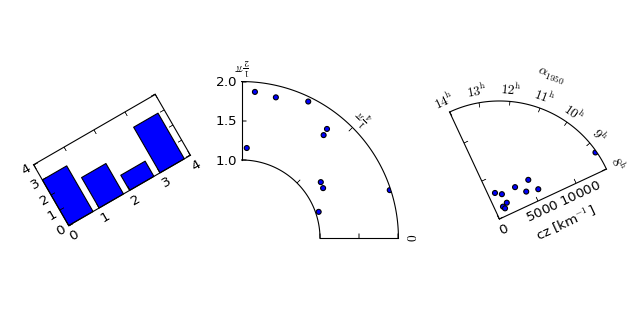# axes_grid example code: demo_floating_axes.py¶from matplotlib.transforms import Affine2D

import mpl_toolkits.axisartist.floating_axes as floating_axes

import numpy as np
import  mpl_toolkits.axisartist.angle_helper as angle_helper
from matplotlib.projections import PolarAxes
from mpl_toolkits.axisartist.grid_finder import FixedLocator, MaxNLocator, \
DictFormatter

def setup_axes1(fig, rect):
"""
A simple one.
"""
tr = Affine2D().scale(2, 1).rotate_deg(30)

grid_helper = floating_axes.GridHelperCurveLinear(tr, extremes=(0, 4, 0, 4))

ax1 = floating_axes.FloatingSubplot(fig, rect, grid_helper=grid_helper)

aux_ax = ax1.get_aux_axes(tr)

grid_helper.grid_finder.grid_locator1._nbins = 4
grid_helper.grid_finder.grid_locator2._nbins = 4

return ax1, aux_ax

def setup_axes2(fig, rect):
"""
With custom locator and formatter.
Note that the extreme values are swapped.
"""

#tr_scale = Affine2D().scale(np.pi/180., 1.)

tr = PolarAxes.PolarTransform()

pi = np.pi
angle_ticks = [(0, r"$0$"),
(.25*pi, r"$\frac{1}{4}\pi$"),
(.5*pi, r"$\frac{1}{2}\pi$")]
grid_locator1 = FixedLocator([v for v, s in angle_ticks])
tick_formatter1 = DictFormatter(dict(angle_ticks))

grid_locator2 = MaxNLocator(2)

grid_helper = floating_axes.GridHelperCurveLinear(tr,
extremes=(.5*pi, 0, 2, 1),
grid_locator1=grid_locator1,
grid_locator2=grid_locator2,
tick_formatter1=tick_formatter1,
tick_formatter2=None,
)

ax1 = floating_axes.FloatingSubplot(fig, rect, grid_helper=grid_helper)

# create a parasite axes whose transData in RA, cz
aux_ax = ax1.get_aux_axes(tr)

aux_ax.patch = ax1.patch # for aux_ax to have a clip path as in ax
ax1.patch.zorder=0.9 # but this has a side effect that the patch is
# drawn twice, and possibly over some other
# artists. So, we decrease the zorder a bit to
# prevent this.

return ax1, aux_ax

def setup_axes3(fig, rect):
"""
Sometimes, things like axis_direction need to be adjusted.
"""

# rotate a bit for better orientation
tr_rotate = Affine2D().translate(-95, 0)

tr_scale = Affine2D().scale(np.pi/180., 1.)

tr = tr_rotate + tr_scale + PolarAxes.PolarTransform()

grid_locator1 = angle_helper.LocatorHMS(4)
tick_formatter1 = angle_helper.FormatterHMS()

grid_locator2 = MaxNLocator(3)

ra0, ra1 = 8.*15, 14.*15
cz0, cz1 = 0, 14000
grid_helper = floating_axes.GridHelperCurveLinear(tr,
extremes=(ra0, ra1, cz0, cz1),
grid_locator1=grid_locator1,
grid_locator2=grid_locator2,
tick_formatter1=tick_formatter1,
tick_formatter2=None,
)

ax1 = floating_axes.FloatingSubplot(fig, rect, grid_helper=grid_helper)

ax1.axis["left"].set_axis_direction("bottom")
ax1.axis["right"].set_axis_direction("top")

ax1.axis["bottom"].set_visible(False)
ax1.axis["top"].set_axis_direction("bottom")
ax1.axis["top"].toggle(ticklabels=True, label=True)
ax1.axis["top"].major_ticklabels.set_axis_direction("top")
ax1.axis["top"].label.set_axis_direction("top")

ax1.axis["left"].label.set_text(r"cz [km$^{-1}$]")
ax1.axis["top"].label.set_text(r"$\alpha_{1950}$")

# create a parasite axes whose transData in RA, cz
aux_ax = ax1.get_aux_axes(tr)

aux_ax.patch = ax1.patch # for aux_ax to have a clip path as in ax
ax1.patch.zorder=0.9 # but this has a side effect that the patch is
# drawn twice, and possibly over some other
# artists. So, we decrease the zorder a bit to
# prevent this.

return ax1, aux_ax

if 1:
import matplotlib.pyplot as plt
fig = plt.figure(1, figsize=(8, 4))

ax1, aux_ax2 = setup_axes1(fig, 131)
aux_ax2.bar([0, 1, 2, 3], [3, 2, 1, 3])

#theta = np.random.rand(10) #*.5*np.pi

ax2, aux_ax2 = setup_axes2(fig, 132)

theta = np.random.rand(10)*.5*np.pi